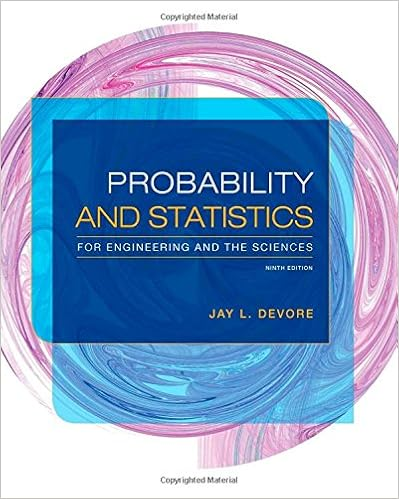# Group Work Answers 11 - The following data represents 20...

• Lab Report
• kwin30
• 3
• 100% (6) 6 out of 6 people found this document helpful

This preview shows page 1 - 3 out of 3 pages.

##### We have textbook solutions for you!
The document you are viewing contains questions related to this textbook.The document you are viewing contains questions related to this textbook.
Chapter 13 / Exercise 13
Probability and Statistics for Engineering and the Sciences
DevoreExpert Verified##### We have textbook solutions for you!
The document you are viewing contains questions related to this textbook.The document you are viewing contains questions related to this textbook.
Chapter 13 / Exercise 13
Probability and Statistics for Engineering and the Sciences
DevoreExpert Verified
The following data represents 20 random samples from a discrete uniform distribution S={1,2,3,4,5,6,7,8,9}. The sample mean ()Xwas calculated for each group: 1.Consider the sample mean as a random variable (the last column) and group the data into the following categories and make a histogram:
.2
.2
1
2.Describe the shape of the data Mound shaped, clustered to center. 3.Calculate the mean of these 20 values and =use the Range/4 to estimate the standard deviation.
4.For this discrete uniform distribution, μ= 5 and σ= 2.58. Based on the Central Limit Theorem, what would the mean and standard deviation of the sample mean random variable be? How does this compare with results from question 3?
•••